SSC (English Medium) Class 10th Board ExamMaharashtra State Board
Share

# Balbharati solutions for Class 10th Board Exam Geometry chapter 3 - Circle

## Balbharati Class 10 Mathematics 2 Geometry

#### Balbharati Balbharati Class 10 Mathematics 2 Geometry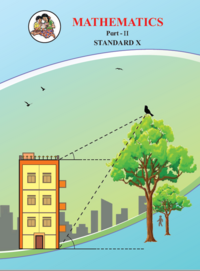## Chapter 3: Circle

#### Chapter 3: Circle solutions [Page 55]

Q 1 | Page 55

In the adjoining figure the radius of a circle with centre C is 6 cm, line AB is a tangent at A. Answer the following questions.
(1) What is the measure of ∠CAB ? Why ?
(2) What is the distance of point C from line AB? Why ?
(3) d(A,B) = 6 cm, find d(B,C).
(4) What is the measure of ∠ABC ? Why ?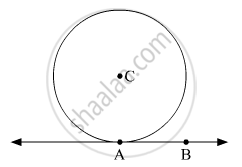Q 2 | Page 55

In the adjoining figure, O is the centre of the circle. From point R, seg RM and seg RN are tangent segments touching the circle at M and N. If (OR) = 10 cm and radius of the circle = 5 cm, then
(1) What is the length of each tangent segment ?
(2) What is the measure of ∠MRO ?
(3) What is the measure of ∠ MRN ?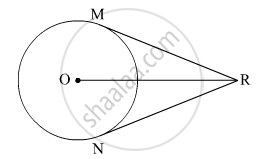Q 3 | Page 55

Seg RM and seg RN are tangent segments of a circle with centre O. Prove that seg OR bisects ∠MRN as well as ∠MON.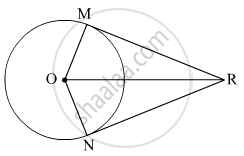Q 4 | Page 55

What is the distance between two parallel tangents of a circle having radius 4.5 cm ? Justify your answer.

#### Chapter 3: Circle solutions [Page 58]

Q 1 | Page 58

Two circles having radii 3.5 cm and 4.8 cm touch each other internally. Find the distance between their centres.

Q 2 | Page 58

Two circles of radii 5.5 cm and 4.2 cm touch each other externally. Find the distance between their centres.

Q 3 | Page 58

If radii of two circles are 4 cm and 2.8 cm. Draw figure of these circles touching each other – (i) externally (ii) internally.

Q 4 | Page 58

In the given figure, the circles with centres P and Q touch each other at R. A line passing through R meets the circles at A and B respectively. Prove that – (1) seg AP || seg BQ,
(2) ∆APR ~ ∆RQB, and
(3) Find ∠ RQB if ∠ PAR = 35°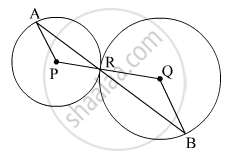Q 5 | Page 58

In the given figure, the circles with centres A and B touch each other at E. Line is a common tangent which touches the circles at C and D respectively. Find the length of seg CD if the radii of the circles are 4 cm, 6 cm.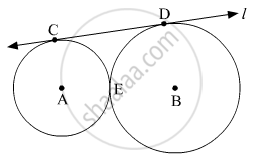#### Chapter 3: Circle solutions [Pages 63 - 64]

Q 1 | Page 63

In the given figure, points G, D, E, F are concyclic points of a circle with centre C.
∠ ECF = 70°, m(arc DGF) = 200° find m(arc DE) and m(arc DEF).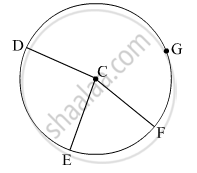Q 2 | Page 64

In the given figure, ∆QRS is an equilateral triangle. Prove that,
(1) arc RS ≅ arc QS ≅ arc QR
(2) m(arc QRS) = 240°.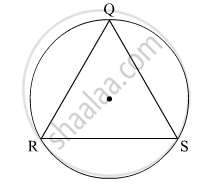Q 3 | Page 64

In the given figure, chord AB ≅ chord CD, Prove that, arc AC ≅ arc BD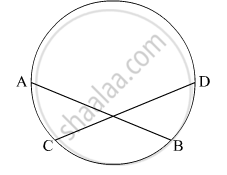#### Chapter 3: Circle solutions [Pages 73 - 74]

Q 1 | Page 73

In the given figure, in a circle with centre O, length of chord AB is equal to the radius of the circle. Find measure of each of the following.
(1) ∠ AOB (2)∠ ACB
(3) arc AB (4) arc ACB.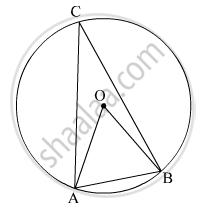Q 2 | Page 73

In the given figure, ▢PQRS is cyclic. side PQ ≅ side RQ. ∠ PSR = 110°, Find–
(1) measure of ∠ PQR
(2) m(arc PQR)
(3) m(arc QR)
(4) measure of ∠ PRQ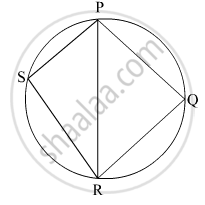Q 3 | Page 73

▢MRPN is cyclic, ∠ R = (5– 13)°, ∠ N = (4+ 4)°. Find measures of ∠ R and ∠ N.

Q 4 | Page 73

In the given figure, seg RS is a diameter of the circle with centre O. Point T lies in the exterior of the circle. Prove that ∠ RTS is an acute angle.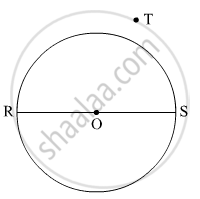Q 5 | Page 73

Prove that, any rectangle is a cyclic quadrilateral.

Q 6 | Page 74

In the given figure, altitudes YZ and XT of ∆WXY intersect at P. Prove that,
(1) ▢WZPT is cyclic.
(2) Points X, Z, T, Y are concyclic.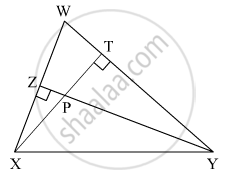Q 7 | Page 74

In the given figure, m(arc NS) = 125°, m(arc EF) = 37°, find the measure ∠ NMS.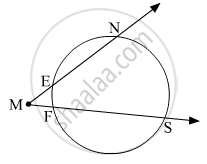Q 8 | Page 74

In the given figure, chords AC and DE intersect at B. If ∠ ABE = 108°, m(arc AE) = 95°, find m(arc DC).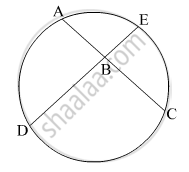#### Chapter 3: Circle solutions [Page 82]

Q 1 | Page 82

In the given figure, ray PQ touches the circle at point Q. PQ = 12, PR = 8, find PS and RS.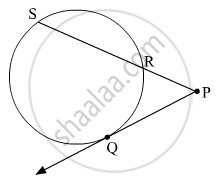Q 2 | Page 82

In the given figure, chord MN and chord RS intersect at point D.
(1) If RD = 15, DS = 4,
MD = 8 find DN
(2) If RS = 18, MD = 9,
DN = 8 find DS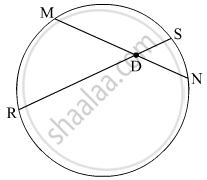Q 3 | Page 82

In the given figure, O is the centre of the circle and B is a point of contact. seg OE ⊥ seg AD, AB = 12, AC = 8, find
(1) AD (2) DC (3) DE.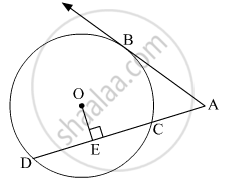Q 4 | Page 82

In the given figure, if PQ = 6, QR = 10, PS = 8 find TS.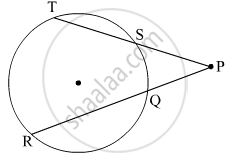Q 5 | Page 82

In the given figure, seg EF is a diameter and seg DF is a tangent segment. The radius of the circle is r. Prove that, DE × GE = 4r2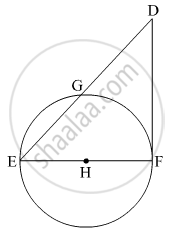#### Chapter 3: Circle solutions [Pages 82 - 84]

Q 1.01 | Page 83

Four alternative answers for the following question is given. Choose the correct alternative.
(1) Two circles of radii 5.5 cm and 3.3 cm respectively touch each other. What is the distance between their centers ?

(A) 4.4 cm (B) 8.8 cm (C) 2.2 cm (D) 8.8 or 2.2 cm
Q 1.02 | Page 82

Four alternative answers for the following question is given. Choose the correct alternative.
Two circles intersect each other such that each circle passes through the centre of the other. If the distance between their centres is 12, what is the radius of each circle ?

(A) 6 cm (B) 12 cm (C) 24 cm (D) can’t say
Q 1.03 | Page 83

Four alternative answers for the following question is given. Choose the correct alternative.
A circle touches all sides of a parallelogram. So the parallelogram must be a, ................... .

(A) rectangle (B) rhombus (C) square (D) trapezium
Q 1.04 | Page 83

Four alternative answers for the following question is given. Choose the correct alternative.
Length of a tangent segment drawn from a point which is at a distance 12.5 cm from the centre of a circle is 12 cm, find the diameter of the circle.

(A) 25 cm (B) 24 cm (C) 7 cm (D) 14 cm
Q 1.05 | Page 83

Four alternative answers for the following question is given. Choose the correct alternative.
If two circles are touching externally, how many common tangents of them can be drawn?

(A) One (B) Two (C) Three (D) Four
Q 1.06 | Page 83

Four alternative answers for the following question is given. Choose the correct alternative.
∠ACB is inscribed in arc ACB of a circle with centre O. If ∠ACB = 65°, find m(arc ACB).

(A) 65° (B) 130° (C) 295° (D) 230°
Q 1.07 | Page 83

Four alternative answers for the following question is given. Choose the correct alternative.
Chords AB and CD of a circle intersect inside the circle at point E. If AE = 5.6, EB = 10, CE = 8, find ED.

(A) 7 (B) 8 (C) 11.2 (D) 9
Q 1.08 | Page 83

Four alternative answers for the following question is given. Choose the correct alternative.
In a cyclic ▢ABCD, twice the measure of ∠A is thrice the measure of ∠C. Find the measure of ∠C?

(A) 36 (B) 72 (C) 90 (D) 108
Q 1.09 | Page 83

Four alternative answers for the following question is given. Choose the correct alternative.
Points A, B, C are on a circle, such that m(arc AB) = m(arc BC) = 120°. No point, except point B, is common to the arcs. Which is the type of ∆ABC?

(A) Equilateral triangle (B) Scalene triangle (C) Right angled triangle (D) Isosceles triangle
Q 1.1 | Page 83

Four alternative answers for the following question is given. Choose the correct alternative.

(10) Seg XZ is a diameter of a circle. Point Y lies in its interior. How many of the following statements are true ? (i) It is not possible that ∠XYZ is an acute angle. (ii) ∠XYZ can’t be a right angle. (iii) ∠XYZ is an obtuse angle. (iv) Can’t make a definite statement for measure of ∠XYZ.

(A) Only one (B) Only two (C) Only three (D) All
Q 2 | Page 84

Line touches a circle with centre O at point P. If radius of the circle is 9 cm, answer the following.
(1) What is d(O, P) = ? Why ?
(2) If d(O, Q) = 8 cm, where does the point Q lie ?
(3) If d(OQ) = 15 cm, How many locations of point Q are line on line l? At what distance will each of them be from point P?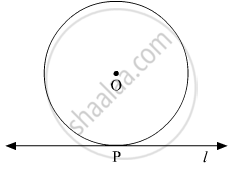Q 3 | Page 84

In the given figure, M is the centre of the circle and seg KL is a tangent segment.
If MK = 12, KL = $6\sqrt{3}$ then find –
(2) Measures of ∠K and ∠M.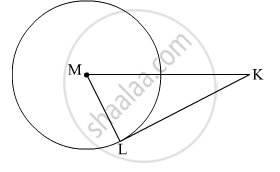Q 4 | Page 84

In the given figure, O is the centre of the ci
rcle. Seg AB, seg AC are tangent segments. Radius of the circle is and l(AB) = , Prove that, ▢ABOC is a square.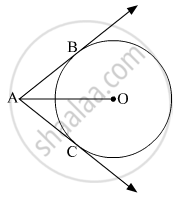## Chapter 3: Circle

#### Balbharati Balbharati Class 10 Mathematics 2 Geometry## Balbharati solutions for Class 10th Board Exam Geometry chapter 3 - Circle

Balbharati solutions for Class 10th Board Exam Geometry chapter 3 (Circle) include all questions with solution and detail explanation. This will clear students doubts about any question and improve application skills while preparing for board exams. The detailed, step-by-step solutions will help you understand the concepts better and clear your confusions, if any. Shaalaa.com has the Maharashtra State Board Balbharati Class 10 Mathematics 2 Geometry solutions in a manner that help students grasp basic concepts better and faster.

Further, we at Shaalaa.com provide such solutions so that students can prepare for written exams. Balbharati textbook solutions can be a core help for self-study and acts as a perfect self-help guidance for students.

Concepts covered in Class 10th Board Exam Geometry chapter 3 Circle are Theorem - Converse of Tangent at Any Point to the Circle is Perpendicular to the Radius, Number of Tangents from a Point to a Circle, Inscribed Angle Theorem, Intercepted Arc, Inscribed Angle, Property of Sum of Measures of Arcs, Tangent Segment Theorem, Converse of Tangent Theorem, Circles passing through one, two, three points, Theorem of External Division of Chords, Theorem of Internal Division of Chords, Converse of Theorem of the Angle Between Tangent and Secant, Theorem of Angle Between Tangent and Secant, Converse of Cyclic Quadrilateral Theorem, Corollary of Cyclic Quadrilateral Theorem, Theorem of Cyclic Quadrilateral, Corollaries of Inscribed Angle Theorem, Angle Subtended by the Arc to the Point on the Circle, Angle Subtended by the Arc to the Centre, Introduction to an Arc, Touching Circles, Number of Tangents from a Point on a Circle, Tangent to a Circle, Tangents and Its Properties, Tangent Properties - If Two Circles Touch, the Point of Contact Lies on the Straight Line Joining Their Centers, Cyclic Properties, Tangent - Secant Theorem, Cyclic Quadrilateral.

Using Balbharati Class 10th Board Exam solutions Circle exercise by students are an easy way to prepare for the exams, as they involve solutions arranged chapter-wise also page wise. The questions involved in Balbharati Solutions are important questions that can be asked in the final exam. Maximum students of Maharashtra State Board Class 10th Board Exam prefer Balbharati Textbook Solutions to score more in exam.

Get the free view of chapter 3 Circle Class 10th Board Exam extra questions for Geometry and can use Shaalaa.com to keep it handy for your exam preparation

S